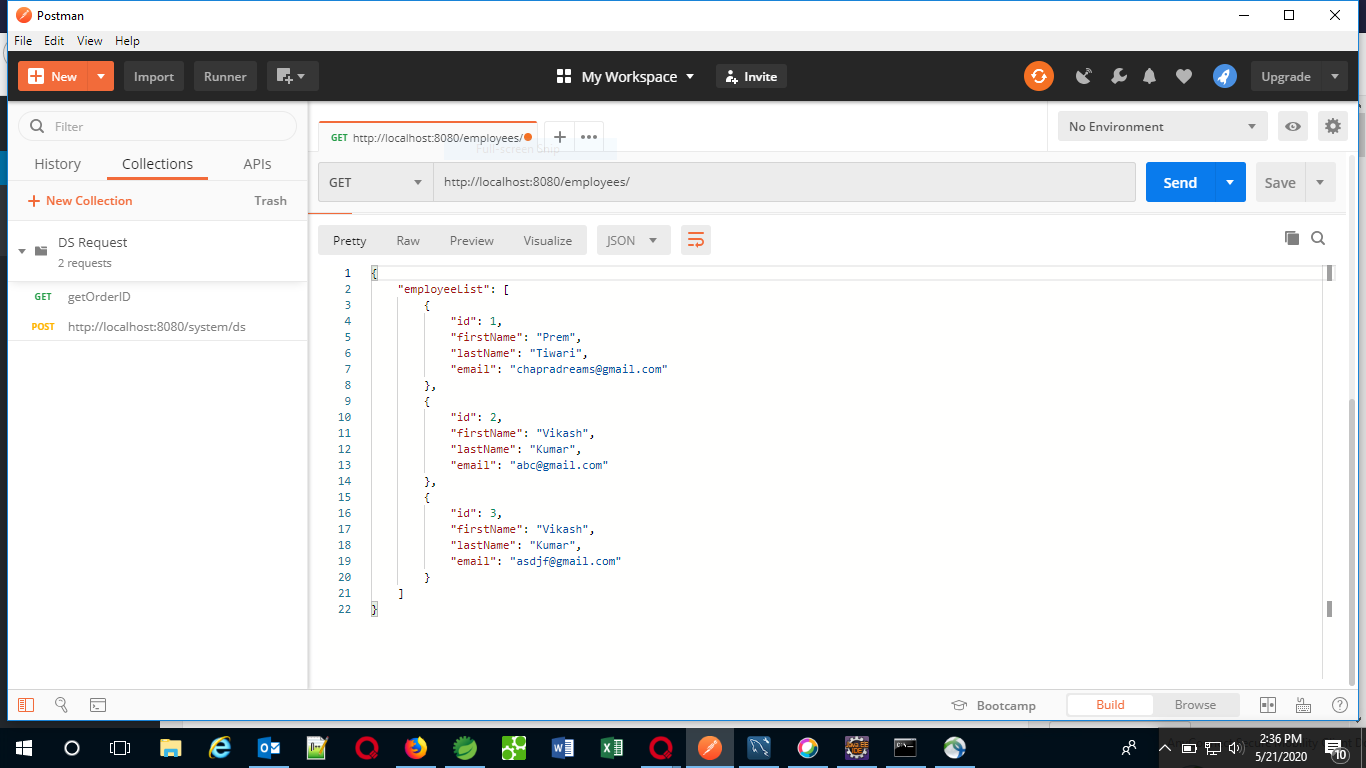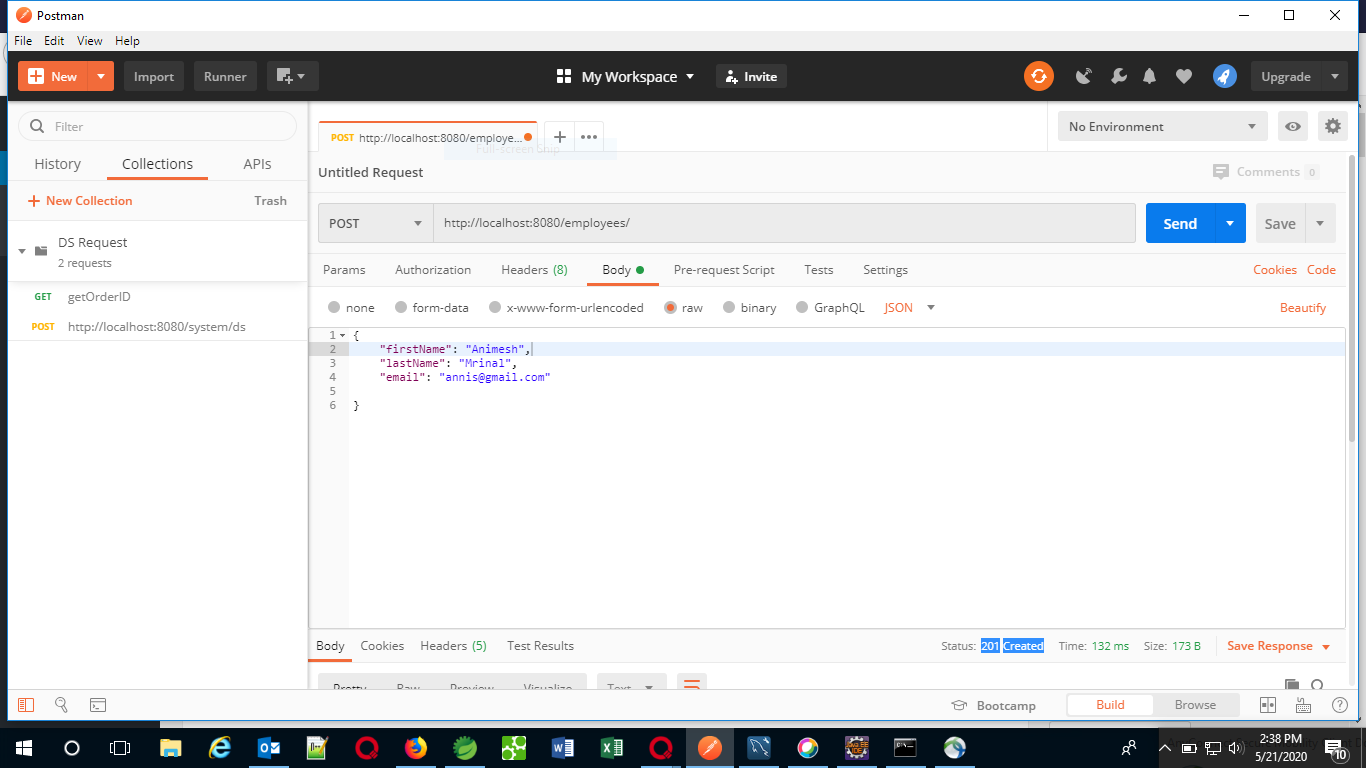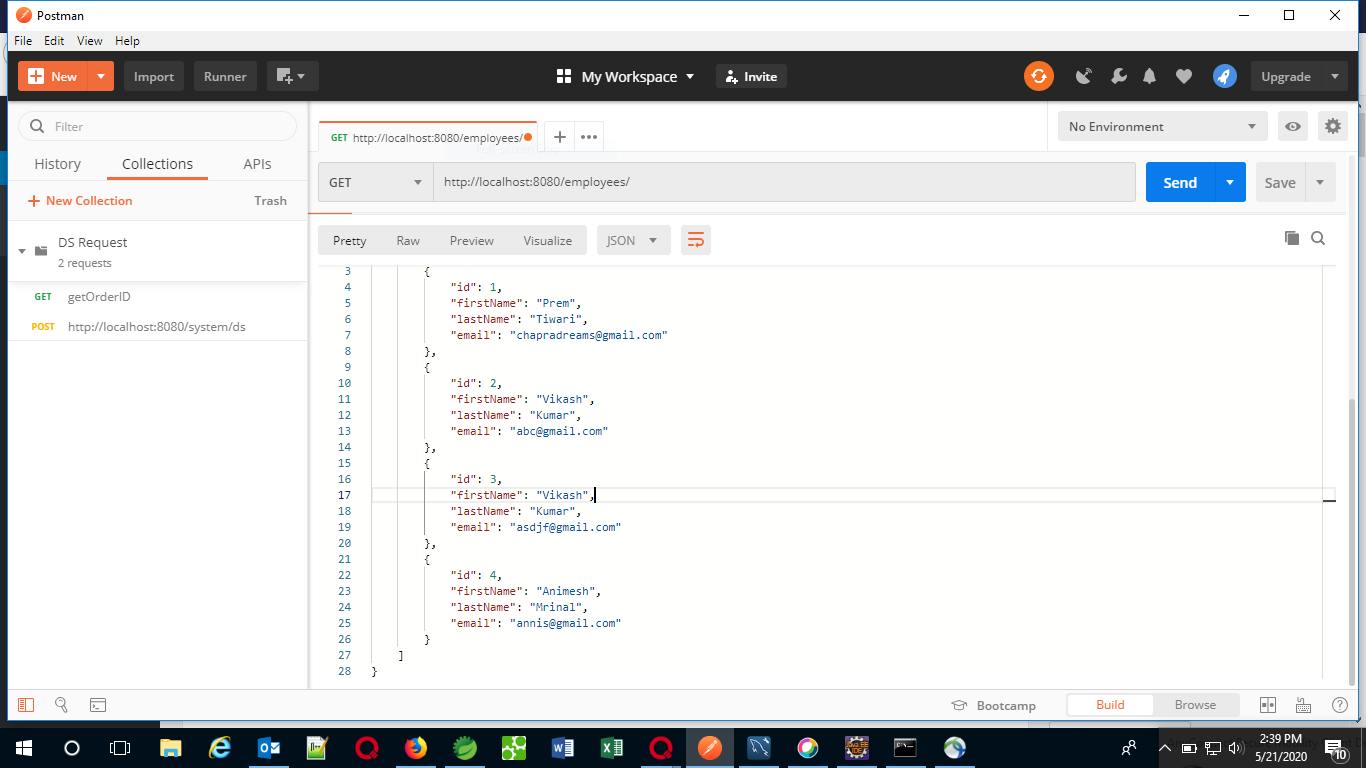GFG App
Open AppBrowser
Continue

# How to create a REST API using Java Spring Boot

Representational state transfer (REST) is a software architectural style that defines a set of constraints to be used for creating Web services. Web services that conform to the REST architectural style, called RESTful Web services, provide interoperability between computer systems on the Internet. RESTful Web services allow the requesting systems to access and manipulate textual representations of Web resources by using a uniform and predefined set of stateless operations. Other kinds of Web services, such as SOAP Web services, expose their own arbitrary sets of operations.

In this article, we will understand how to create a rest API using spring boot.

Spring is widely used for creating scalable applications. For web applications, Spring provides Spring MVC which is a widely used module of spring which is used to create scalable web applications. But the main disadvantage of spring projects is that configuration is really time-consuming and can be a bit overwhelming for the new developers. The solution to this is Spring Boot. Spring Boot is built on the top of the spring and contains all the features of spring. In this article, we will create a REST API to add employees to the employee list and get the list of employees. In order to do this, we first have to create a simple Spring Boot project in any of the IDE’s and follow the steps:

1. Initially, we need to define the employee entity. Therefore, the following employee class is defined:

 `package` `com.example.demo; ` ` `  `// Creating an entity Employee ` `public` `class` `Employee { ` ` `  `    ``public` `Employee() {} ` ` `  `    ``// Parameterized Constructor ` `    ``// to assign the values ` `    ``// to the properties of ` `    ``// the entity ` `    `` ``public` `Employee( ` `        ``Integer id, String firstName, ` `        ``String lastName, String email) ` `    ``{ ` ` `  `        ``super``(); ` ` `  `        ``this``.id = id; ` ` `  `        ``this``.firstName = firstName; ` ` `  `        ``this``.lastName = lastName; ` ` `  `        ``this``.email = email; ` ` `  `        ``    ` `    ``} ` ` `  `    ``   ``private` `Integer id; ` ` `  `    ``   ``private` `String firstName; ` ` `  `    ``   ``private` `String lastName; ` ` `  `    ``   ``private` `String email; ` ` `  `    ``// Overriding the toString method ` `    ``// to find all the values ` `    ``@Override` `   ``public` `String toString() ` `    ``{ ` ` `  `        ``return` `"Employee [id="` `            ``+ id + ``", firstName="` `            ``+ firstName + ``", lastName="` `            ``+ lastName + ``", email="` `            ``+ email + ``"]"``; ` ` `  `        ``    ` `    ``} ` ` `  `    ``// Getters and setters of ` `    ``// the properties ` `    ``public` `Integer getId() ` `    ``{ ` ` `  `        `` ``return` `id; ` `    ``} ` ` `  `    ``public` `void` `setId(Integer id) ` `    ``{ ` ` `  `        `` ``this``.id = id; ` `    ``} ` ` `  `    ``public` `String getFirstName() ` `    ``{ ` ` `  `        `` ``return` `firstName; ` `    ``} ` ` `  `    ``public` `void` `setFirstName( ` `        ``String firstName) ` `    ``{ ` ` `  `        `` ``this``.firstName = firstName; ` `    ``} ` ` `  `    ``public` `String getLastName() ` `    ``{ ` ` `  `        `` ``return` `lastName; ` `    ``} ` ` `  `    ``public` `void` `setLastName( ` `        ``String lastName) ` `    ``{ ` ` `  `        `` ``this``.lastName = lastName; ` `    ``} ` ` `  `    ``public` `String getEmail() ` `    ``{ ` ` `  `        `` ``return` `email; ` `    ``} ` ` `  `    ``public` `void` `setEmail(String email) ` `    ``{ ` ` `  `        `` ``this``.email = email; ` `    ``} ` `} `

2. Now, we need to create a storage class which stores the list of all the employees:

 `package` `com.example.demo; ` ` `  `import` `java.util.ArrayList; ` `import` `java.util.List; ` ` `  `// Class to store the list of ` `// all the employees in an ` `// Array List ` `public` `class` `Employees { ` ` `  `    ``private` `List employeeList; ` ` `  `    ``// Method to return the list ` `    ``// of employees ` `    ``public` `List getEmployeeList() ` `    ``{ ` ` `  `        ``if` `(employeeList == ``null``) { ` ` `  `            ``employeeList ` `                ``= ``new` `ArrayList<>(); ` ` `  `            ``        ` `        ``} ` ` `  `        ``return` `employeeList; ` ` `  `        ``    ` `    ``} ` ` `  `    ``public` `void` `    ``setEmployeeList( ` `        ``List employeeList) ` `    ``{ ` `        ``this``.employeeList ` `            ``= employeeList; ` `    ``} ` `} `

3. Till now, we have defined the entity employee and created a storage class. Now, we need to access the employees. So, we create a class from where we will create an object of the storage class to store the employees:

 `package` `com.example.demo; ` ` `  `import` `org.springframework ` `    ``.stereotype ` `    ``.Repository; ` ` `  `// Importing the employees class to ` `// use the defined properties ` `// in this class ` `import` `com.example.demo.Employees; ` ` `  `@Repository` ` `  `// Class to create a list ` `// of employees ` `public` `class` `EmployeeDAO { ` ` `  `    ``private` `static` `Employees list ` `        ``= ``new` `Employees(); ` ` `  `    ``// This static block is executed ` `    ``// before executing the main ` `    ``// block ` `    ``static` `    ``{ ` ` `  `        ``// Creating a few employees ` `        ``// and adding them to the list ` `        ``list.getEmployeeList().add( ` `            ``new` `Employee( ` `                ``1``, ` `                ``"Prem"``, ` `                ``"Tiwari"``, ` `                ``"chapradreams@gmail.com"``)); ` ` `  `        ``list.getEmployeeList().add( ` `            ``new` `Employee( ` `                ``2``, ``"Vikash"``, ` `                ``"Kumar"``, ` `                ``"abc@gmail.com"``)); ` ` `  `        ``list.getEmployeeList().add( ` `            ``new` `Employee( ` `                ``3``, ``"Ritesh"``, ` `                ``"Ojha"``, ` `                ``"asdjf@gmail.com"``)); ` ` `  `        ``    ` `    ``} ` ` `  `    ``// Method to return the list ` `    ``public` `Employees getAllEmployees() ` `    ``{ ` ` `  `        ``return` `list; ` `    ``} ` ` `  `    ``    ` `        ``// Method to add an employee ` `        ``// to the employees list ` `        ``public` `void` `        ``addEmployee(Employee employee) ` `    ``{ ` `        ``list.getEmployeeList() ` `            ``.add(employee); ` `        ``    ` `    ``} ` `} `

4. Finally, we need to create a controller class which is the actual implementation of the REST API. According to the REST rules, every new entry in the database has to be called by the POST method and all the requests from the database must be called using the GET method. The same methods are implemented in the following code:

 `package` `com.example.demo; ` ` `  `import` `java.net.URI; ` `import` `org.springframework.beans ` `    ``.factory.annotation.Autowired; ` `import` `org.springframework.http ` `    ``.ResponseEntity; ` `import` `org.springframework.web.bind ` `    ``.annotation.GetMapping; ` `import` `org.springframework.web.bind ` `    ``.annotation.PostMapping; ` `import` `org.springframework.web.bind ` `    ``.annotation.RequestBody; ` `import` `org.springframework.web.bind ` `    ``.annotation.RequestMapping; ` `import` `org.springframework.web.bind ` `    ``.annotation.RestController; ` `import` `org.springframework.web.servlet ` `    ``.support.ServletUriComponentsBuilder; ` ` `  `// Import the above-defined classes ` `// to use the properties of those ` `// classes ` `import` `com.example.demo.Employees; ` `import` `com.example.demo.EmployeeDAO; ` `import` `com.example.demo.Employee; ` ` `  `// Creating the REST controller ` `@RestController` `@RequestMapping``(path = ``"/employees"``) ` `public` `class` `EmployeeController { ` ` `  `    ``@Autowired` `   ``private` `EmployeeDAO employeeDao; ` `    ``    ` `        ``// Implementing a GET method ` `        ``// to get the list of all ` `        ``// the employees ` `   ``@GetMapping``( ` `        ``path = ``"/"``, ` `        ``produces = ``"application/json"``) ` ` `  `    ``public` `Employees getEmployees() ` `    ``{ ` ` `  `        ``return` `employeeDao ` `            ``.getAllEmployees(); ` `    ``} ` ` `  `    ``    ` `        ``// Create a POST method ` `        ``// to add an employee ` `        ``// to the list ` `   ``@PostMapping``( ` `        ``path = ``"/"``, ` `        ``consumes = ``"application/json"``, ` `        ``produces = ``"application/json"``) ` ` `  `    ``public` `ResponseEntity

5. After implementing all the classes in the project, run the project as Spring Boot App. Once the server starts running, we can send the requests through the browser or postman. We can access the running app by going into the following URL:
```http://localhost:8080/employees/
```

Output: The following is the output generated on running the above project:

1. When a GET request is performed:2. When a POST request is performed:3. Again hitting the GET request after performing the POST request:My Personal Notes arrow_drop_up
Related Tutorials